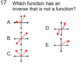# 25 Socrative High School Math Assignments (Part 13) in PDF FilesSubject
Resource Type
File Type

Zip

(73 MB|50 pages)
Also included in:
1. Included in this zip folder are 75 high school math assignments on PDF files. These assignments can be completed at socrative.com or be printed. Each is on 1 page for easy printing. The 1st assignment is free in the preview section. A brief description and title of each:Part 13(1) 2 Way Frequency Ta
\$12.00
\$10.00
Save \$2.00
2. These are great distance learning assignments.These are PDF assignments for Algebra, Geometry and Pre-Calculus. The assignments can be printed or done at Socrative.com. The share numbers are included.Part 1(1) 2 Way Frequency Tables Assignment #1BReading a 2 way table and probability(2) 2 Way Freque
\$96.00
\$70.00
Save \$26.00
Product Description
Included in this zip folder are 25 high school math assignments in PDF files. These assignments can be completed at socrative.com or be printed. Each is on 1 or 2 pages for easy printing. A brief description and title of each:

(1) 2 Way Frequency Tables Assignment #3 (24 questions)
This is a short answer assignment. The student finds missing values and 2 way tables. Some probability questions are asked.

(2) Advanced Factoring Assignment A (24 questions)
Recognize the sum and difference of 2 cubes as well as the difference of 2 squares

(3) Analyze a Parent Function Assignment B (12 questions)
This is a matching assignment. The student matches the graphs to its parent function. The functions are: y=lnx, y=sinx, y=cosx, y=x, y=x^2, y=x^3, y=intx, y=absx, y=e^x, y=1/(1+e^x), y=1/x and y=sqrtx.

(4) Analyze a Parent Function Assignment C (12 questions)
This is a matching assignment. The student matches the graphs to its parent function. The functions are: y=lnx, y=sinx, y=cosx, y=x, y=x^2, y=x^3, y=intx, y=absx, y=e^x, y=1/(1+e^x), y=1/x and y=sqrtx.

(5) Complex Numbers and DeMoivre's Theorem Assignment #1 (24 questions)
Recognize Trig and Standard forms and graph points on the complex plane

(6) Complex Numbers and DeMoivre's Theorem Assignment #2 (24 questions)
Finding roots of complex numbers

(7) Congruence Statements Assignment #1 (24 questions)
Write congruence stalemates in Geometry using symbols and definitions such as median, altitude and bisector

(8) Congruent Triangles Assignment A (15 questions)
Recognize congruence sequences such as AAS and ASA

(9) End Behavior Assignment #3(20 questions)
Finding left and right end behavior of functions

(10) Function Values Assignment #4 (24 questions)
Find function values from graphs, tables and rules. Composite and inverse function values are included

(11) Function Values Assignment #5 (24 questions)
Find function values from graphs, tables and rules. Composite and inverse function values are included

(12) Functions Assessment Assignment #1(40 questions)
A comprehensive review of functions including end behavior, odd and even, limits of discontinuity, function values, boundedness, domain and range

(13) Functions Assessment Assignment #2(40 questions)
A comprehensive review of functions including end behavior, odd and even, limits of discontinuity, function values, boundedness, domain and range

(14) Functions Assessment Assignment #3(40 questions)
A comprehensive review of functions including end behavior, odd and even, limits of discontinuity, function values, boundedness, domain and range

(15) Geometry Definitions Assignment #1 (32 questions)
Many definitions in Geometry are covered

(16) Geometry Definitions Assignment #3 (24 questions)
Many definitions in Geometry are covered

(17) Geometry Definitions Assignment #4 (24 questions)
Many definitions in Geometry are covered

(18) Geometry Definitions Assignment #6 (40 questions)
Many definitions in Geometry are covered

(19) Parabola Unit Assignment #4 (24 questions)
Finding the vertex in vertex form

(20) Parabola Unit Assignment #5 (24 questions)
Finding the vertex in different forms

(21) Parabola Unit Assignment #6 (20 questions)
Use transformational and vertex forms to write equations

(22) Parabola Unit Assignment #8 (24 questions)
Finding the vertex, focus and focal width endpoints of a parabola

(23) Parallel Lines Assignment #3A (15 questions)
Using and not using alternate interior, consecutive interior, corresponding and alternate exterior based upon lines being parallel or not parallel

(24) Parallel Lines Assignment #3B (15 questions)
Using and not using alternate interior, consecutive interior, corresponding and alternate exterior based upon lines being parallel or not parallel

(25) Parallel Lines Assignment #3E (15 questions)
Using and not using alternate interior, consecutive interior, corresponding and alternate exterior based upon lines being parallel or not parallel

Thank you for looking
Total Pages
50 pages
Included
Teaching Duration
N/A
Report this Resource to TpT
Reported resources will be reviewed by our team. Report this resource to let us know if this resource violates TpT’s content guidelines.